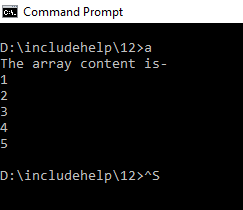# fwrite() function in C language with Example

Here, we are going to learn about the fwrite() function of library header stdio.h in C language with its syntax, example.
Submitted by Souvik Saha, on January 24, 2019

## fwrite() function in C

Prototype:

```    size_t fwrite(void *buffer, size_t length, size_t count, FILE *filename);
```

Parameters:

```    void *buffer, size_t length, size_t count, FILE *filename
```

Return type: size_t

Use of function:

The prototype of the function fwrite() is:

```    size_t fwrite(void *buffer, size_t length, size_t count, FILE *filename);
```

In the file handling, through the fwrite() function we write count number of objects of size length to the input stream filename from the array named as buffer. Its returns the number of objects that it will write to the file. If fewer number of objects will be written or EOF is encountered then it will throw an error.

## fwrite() example in C

```#include <stdio.h>
#include <stdlib.h>

int main()
{
FILE* f;
//initialize the arr1 with values
int arr1 = { 1, 2, 3, 4, 5 };
int arr2;
int i = 0;

//open the file for write operation
if ((f = fopen("includehelp.txt", "w")) == NULL) {
//if the file does not exist print the string
printf("Cannot open the file...");
exit(1);
}
//write the values on the file
if ((fwrite(arr1, sizeof(int), 5, f)) != 5) {
printf("File write error....\n");
}
//close the file
fclose(f);

//open the file for read operation
if ((f = fopen("includehelp.txt", "r")) == NULL) {
//if the file does not exist print the string
printf("Cannot open the file...");
exit(1);
}
//read the values from the file and store it into the array
if ((fread(arr2, sizeof(int), 5, f)) != 5) {
printf("File write error....\n");
}
fclose(f);
for (i = 0; i < 5; i++) {
printf("%d\n", arr2[i]);
}

return 0;
}
```

Output